mscroggs.co.uk
mscroggs.co.uksubscribe

# Blog

2020-05-15
This is a post I wrote for The Aperiodical's Big Lock-Down Math-Off. You can vote for (or against) me here until 9am on Sunday...
Recently, I came across a surprising fact: if you take any quadrilateral and join the midpoints of its sides, then you will form a parallelogram.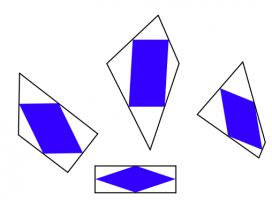The blue quadrilaterals are all parallelograms.
The first thing I thought when I read this was: "oooh, that's neat." The second thing I thought was: "why?" It's not too difficult to show why this is true; you might like to pause here and try to work out why yourself before reading on...
To show why this is true, I started by letting $$\mathbf{a}$$, $$\mathbf{b}$$, $$\mathbf{c}$$ and $$\mathbf{d}$$ be the position vectors of the vertices of our quadrilateral. The position vectors of the midpoints of the edges are the averages of the position vectors of the two ends of the edge, as shown below.
We want to show that the orange and blue vectors below are equal (as this is true of opposite sides of a parallelogram).
We can work these vectors out: the orange vector is$$\frac{\mathbf{d}+\mathbf{a}}2-\frac{\mathbf{a}+\mathbf{b}}2=\frac{\mathbf{d}-\mathbf{b}}2,$$ and the blue vector is$$\frac{\mathbf{c}+\mathbf{d}}2-\frac{\mathbf{b}+\mathbf{c}}2=\frac{\mathbf{d}-\mathbf{b}}2.$$
In the same way, we can show that the other two vectors that make up the inner quadrilateral are equal, and so the inner quadrilateral is a parallelogram.

### Going backwards

Even though I now saw why the surprising fact was true, my wondering was not over. I started to think about going backwards.
It's easy to see that if the outer quadrilateral is a square, then the inner quadrilateral will also be a square.If the outer quadrilateral is a square, then the inner quadrilateral is also a square.
It's less obvious if the reverse is true: if the inner quadrilateral is a square, must the outer quadrilateral also be a square? At first, I thought this felt likely to be true, but after a bit of playing around, I found that there are many non-square quadrilaterals whose inner quadrilaterals are squares. Here are a few: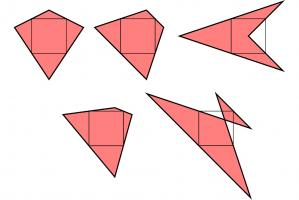A kite, a trapezium, a delta kite, an irregular quadrilateral and a cross-quadrilateral whose innner quadrilaterals are all a square.
There are in fact infinitely many quadrilaterals whose inner quadrilateral is a square. You can explore them in this Geogebra applet by dragging around the blue point:
As you drag the point around, you may notice that you can't get the outer quadrilateral to be a non-square rectangle (or even a non-square parallelogram). I'll leave you to figure out why not...

### Similar postsMathsteroids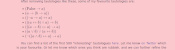Interesting tautologies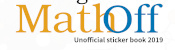Big Internet Math-Off stickers 2019Runge's Phenomenon

Comments in green were written by me. Comments in blue were not written by me.

Allowed HTML tags: <br> <a> <small> <b> <i> <s> <sup> <sub> <u> <spoiler> <ul> <ol> <li>
To prove you are not a spam bot, please type "rebmun" backwards in the box below (case sensitive):
2020-05-03
This is a post I wrote for The Aperiodical's Big Lock-Down Math-Off. You can vote for (or against) me here until 9am on Tuesday...
A few years ago, I made @mathslogicbot, a Twitter bot that tweets logical tautologies.
The statements that @mathslogicbot tweets are made up of variables (a to z) that can be either true or false, and the logical symbols $$\lnot$$ (not), $$\land$$ (and), $$\lor$$ (or), $$\rightarrow$$ (implies), and $$\leftrightarrow$$ (if and only if), as well as brackets. A tautology is a statement that is always true, whatever values are assigned to the variables involved.
To get an idea of how to interpret @mathslogicbot's statements, let's have a look at a few tautologies:
$$( a \rightarrow a )$$. This says "a implies a", or in other words "if a is true, then a is true". Hopefully everyone agrees that this is an always-true statement.
$$( a \lor \lnot a )$$. This says "a or not a": either a is true, or a is not true
$$(a\leftrightarrow a)$$. This says "a if and only if a".
$$\lnot ( a \land \lnot a )$$. This says "not (a and not a)": a and not a cannot both be true.
$$( \lnot a \lor \lnot \lnot a )$$. I'll leave you to think about what this one means.
(Of course, not all statements are tautologies. The statement $$(b\land a)$$, for example, is not a tautology as is can be true or false depending on the values of $$a$$ and $$b$$.)
While looking through @mathslogicbot's tweets, I noticed that a few of them are interesting, but most are downright rubbish. This got me thinking: could I get rid of the bad tautologies like these, and make a list of just the "interesting" tautologies. To do this, we first need to think of different ways tautologies can be bad.
Looking at tautologies the @mathslogicbot has tweeted, I decided to exclude:
• tautologies like $$(a\rightarrow\lnot\lnot\lnot\lnot a)$$ that contain more than one $$\lnot$$ in a row.
• tautologies like $$((a\lor\lnot a)\lor b)$$ that contain a shorter tautology. Instead, tautologies like $$(\text{True}\lor b)$$ should be considered.
• tautologies like $$((a\land\lnot a)\rightarrow b)$$ that contain a shorter contradiction (the opposite of a tautology). Instead, tautologies like $$(\text{False}\rightarrow b)$$ should be considered.
• tautologies like $$(\text{True}\lor\lnot\text{True})$$ or $$((b\land a)\lor\lnot(b\land a)$$ that are another tautology (in this case $$(a\lor\lnot a)$$) with a variable replaced with something else.
• tautologies containing substatements like $$(a\land a)$$, $$(a\lor a)$$ or $$(\text{True}\land a)$$ that are equivalent to just writing $$a$$.
• tautologies that contain a $$\rightarrow$$ that could be replaced with a $$\leftrightarrow$$, because it's more interesting if the implication goes both ways.
• tautologies containing substatements like $$(\lnot a\lor\lnot b)$$ or $$(\lnot a\land\lnot b)$$ that could be replaced with similar terms (in these cases $$(a\land b)$$ and $$(a\lor b)$$ respectively) without the $$\lnot$$s.
• tautologies that are repeats of each other with the order changed. For example, only one of $$(a\lor\lnot a)$$ and $$(\lnot a\lor a)$$ should be included.
After removing tautologies like these, some of my favourite tautologies are:
• $$( \text{False} \rightarrow a )$$
• $$( a \rightarrow ( b \rightarrow a ) )$$
• $$( ( \lnot a \rightarrow a ) \leftrightarrow a )$$
• $$( ( ( a \leftrightarrow b ) \land a ) \rightarrow b )$$
• $$( ( ( a \rightarrow b ) \leftrightarrow a ) \rightarrow a )$$
• $$( ( a \lor b ) \lor ( a \leftrightarrow b ) )$$
• $$( \lnot ( ( a \land b ) \leftrightarrow a ) \rightarrow a )$$
• $$( ( \lnot a \rightarrow b ) \leftrightarrow ( \lnot b \rightarrow a ) )$$
You can find a list of the first 500 "interesting" tautologues here. Let me know on Twitter which is your favourite. Or let me know which ones you think are rubbish, and we can further refine the list...

### Similar postsLogical contradictionsLogic bot, pt. 2Logic botA surprising fact about quadrilaterals

Comments in green were written by me. Comments in blue were not written by me.

Allowed HTML tags: <br> <a> <small> <b> <i> <s> <sup> <sub> <u> <spoiler> <ul> <ol> <li>
To prove you are not a spam bot, please type "h" then "e" then "x" then "a" then "g" then "o" then "n" in the box below (case sensitive):
2019-07-03
This year's Big Internet Math-Off is now underway with 15 completely new contestants (plus one returning contender). As I'm not the returning contestant, I haven't been spending my time preparing my pitches. Instead, I've spent my time making an unofficial Big Internet Math-Off sticker book.
To complete the sticker book, you will need to collect 162 different stickers. Every day, you will be given a pack of 5 stickers; there are also some bonus packs available if you can find them (Hint: keep reading).

### How many stickers will I need?

Using the same method as I did for last year's World Cup sticker book, you can work out that the expected number of stickers needed to finish the sticker book:
If you have already stuck $$n$$ stickers into your album, then the probability that the next sticker you get is new is
$$\frac{162-n}{162}.$$
The probability that the second sticker you get is the next new sticker is
$$\mathbb{P}(\text{next sticker is not new})\times\mathbb{P}(\text{sticker after next is new})$$ $$=\frac{n}{162}\times\frac{162-n}{162}.$$
Following the same method, we can see that the probability that the $$i$$th sticker you buy is the next new sticker is
$$\left(\frac{n}{162}\right)^{i-1}\times\frac{162-n}{162}.$$
Using this, we can calculate the expected number of stickers you will need to buy until you find a new one:
$$\sum_{i=1}^{\infty}i \left(\frac{162-n}{162}\right) \left(\frac{n}{162}\right)^{i-1} = \frac{162}{162-n}$$
Therefore, to get all 162 stickers, you should expect to buy
$$\sum_{n=0}^{161}\frac{162}{162-n} = 918 \text{ stickers}.$$
Using just your daily packs, it will take you until the end of the year to collect this many stickers. Of course, you'll only need to collect this many if you don't swap your duplicate stickers.

### How many stickers will I need if I swap?

To work out the expected number of stickers stickers you'd need if you swap, let's first think about two people who want to complete their stickerbooks together. If there are $$a$$ stickers that both collectors need and $$b$$ stickers that one collector has and the other one needs, then let $$E_{a,b}$$ be the expected number of stickers they need to finish their sticker books. The next sticker they get could be one of three things:
• A sticker they both need (with probability $$\frac{a}{162}$$);
• A sticker one of them needs (with probability $$\frac{b}{162}$$);
• A sticker they both have (with probability $$\frac{162-a-b}{162}$$).
Therefore, the expected number of stickers they need to complete their sticker books is
$$E_{a,b}=1+\frac{a}{162}E_{a-1,b+1}+\frac{b}{162}E_{a,b-1}+\frac{162-a-b}{162}E_{a,b}.$$
This can be rearranged to give
$$E_{a,b}= \frac{162}{a+b}+ \frac{a}{a+b}E_{a-1,b+1} +\frac{b}{a+b}E_{a,b-1}$$
We know that $E_{0,0}=0$ (as if $$a=0$$ and $$b=0$$, both collectors have already finished their sticker books). Using this and the formula above, we can work out that
$$E_{0,1}=162+E_{0,0}=162$$ $$E_{1,0}=162+E_{0,1}=324$$ $$E_{0,2}=\frac{162}2+E_{0,1}=243$$ $$E_{1,1}=\frac{162}2+\frac12E_{0,2}+\frac12E_{1,0}=364.5$$
... and so on until we find that $$E_{162,0}=1269$$, and so our collectors should expect to collect 634 stickers each to complete their sticker books.
For three people, we can work out that if there are $$a$$ stickers that all three need, $$b$$ stickers that two need, and $$c$$ stickers that one needs, then
$$E_{a,b,c} = \frac{162}{a+b+c}+ \frac{a}{a+b+c}E_{a-1,b+1,c} +\frac{b}{a+b+c}E_{a,b-1,c+1} +\frac{c}{a+b+c}E_{a,b,c-1}.$$
In the same way as for two people, we find that $$E_{162,0,0}=1572$$, and so our collectors should expect to collect 524 stickers each to complete their sticker books.
Doing the same thing for four people gives an expected 463 stickers required each.
After four people, however, the Python code I wrote to do these calculations takes too long to run, so instead I approximated the numbers by simulating 500 groups of $$n$$ people collecting stickers, and taking the average number of stickers they needed. The results are shown in the graph below.
The red dots are the expected values we calculated exactly, and the blue crosses are the simulated values. It looks like you'll need to collect at least 250 stickers to finish the album: in order to get this many before the end of the Math-Off, you'll need to find 20 bonus packs...
Of course, these are just the mean values and you could get lucky and need fewer stickers. The next graph shows box plots with the quartiles of the data from the simulations.
So if you're lucky, you could complete the album with fewer stickers or fewer friends.
As a thank you for reading to the end of this blog post, here's a link that will give you two bonus packs and help you on your way to the 250 expected stickers...

### Similar postsA surprising fact about quadrilateralsInteresting tautologiesRunge's PhenomenonWorld Cup stickers 2018, pt. 3

Comments in green were written by me. Comments in blue were not written by me.
Link to sticker book, in the first paragraph, does not work. It points to mathoffstickbook.com
Pat Ashforth
minor typo for the 2 collector case

> and so our collectors should expect to collect 364 stickers

should be 634.

Allowed HTML tags: <br> <a> <small> <b> <i> <s> <sup> <sub> <u> <spoiler> <ul> <ol> <li>
To prove you are not a spam bot, please type "cosine" in the box below (case sensitive):
2019-03-26
I originally wrote this post for The Aperiodical.
A few months ago, Adam Townsend went to lunch and had a conversation. I wasn't there, but I imagine the conversation went something like this:Smitha: Hello.
Smitha: You know how the \hat command in LaTeΧ puts a caret above a letter?... Well I was thinking it would be funny if someone made a package that made the \hat command put a picture of an actual hat on the symbol instead?
Adam: (After a few hours of laughter.) I'll see what my flatmate is up to this weekend...
Jeff: What on Earth are you two talking about?!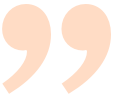As anyone who has been anywhere near maths at a university in the last ∞ years will be able to tell you, LaTeΧ is a piece of maths typesetting software. It's a bit like a version of Word that runs in terminal and makes PDFs with really pretty equations.
By default, LaTeΧ can't do very much, but features can easily added by importing packages: importing the graphicsx package allows you to put images in your PDF; importing geometry allows you to easily change the page margins; and importing realhats makes the \hat command put real hats above symbols.

### Changing the behaviour of \hat

By default, the LaTeΧ command \hat puts a pointy "hat" above a symbol:
After Adam's conversation, we had a go at redefining the \hat command by putting the following at the top of our LaTeΧ file.
LaTeΧ
\renewcommand{\hat}{
% We put our new definition here
}

After a fair amount of fiddling with the code, we eventually got it to produce the following result:a (left) and \hat{a} (right) while using the realhats package
We were now ready to put our code into a package so others could use it.

### How to write a package

A LaTeΧ package is made up of:
• a sty file, containing a collection of commands like the one we wrote above;
• a PDF of documentation showing users how to use your package;
It's quite common to make the first two of these by making a dtx file and an ins file. And no, we have no idea either why these are the file extensions used or why this is supposedly simpler than making a sty file and a PDF.
The ins file says which bits of the dtx should be used to make up the sty file. Our ins file looks like this:
LaTeΧ
\input{docstrip.tex}
\keepsilent
\usedir{tex/latex/realhats}
\preamble
\endpreamble
\generate{
\file{realhats.sty}{\from{realhats.dtx}{realhats}}
}
\endbatchfile
The most important command in this file is \generate: this says that that the file realhats.sty should be made from the file realhats.dtx taking all the lines that are marked as part of realhats. The following is part of our dtx file:
LaTeΧ
%\lstinline{realhats} is a package for \LaTeX{} that makes the \lstinline{\hat}
%command put real hats on symbols.
%For example, the input \lstinline@\hat{a}=\hat{b}@ will produce the output:
%$\hat{a}=\hat{b}$
%To make a vector with a hat, the input \lstinline@\hat{\mathbf{a}}@ produces:
%$\hat{\mathbf{a}}$
%
%\iffalse
%<*documentation>
\documentclass{article}
\usepackage{realhats}
\usepackage{doc}
\usepackage{listings}
\title{realhats}
\begin{document}
\maketitle
\DocInput{realhats.dtx}
\end{document}
%</documentation>
%\fi

%\iffalse
%<*realhats>
\NeedsTeXFormat{LaTeX2e}
\ProvidesPackage{realhats}[2019/02/02 realhats]

\RequirePackage{amsmath}
\RequirePackage{graphicx}
\RequirePackage{ifthen}
\renewcommand{\hat}{
% We put our new definition here
}
%</realhats>
%\fi
The lines near the end between <*realhats> and </realhats> will be included in the sty file, as they are marked at part of realhats.
The rest of this file will make the PDF documentation when the dtx file is compiled. The command \DocInput tells LaTeΧ to include the dtx again, but with the %s that make lines into comments removed. In this way all the comments that describe the functionality will end up in the PDF. The lines that define the package will not be included in the PDF as they are between \iffalse and \fi.
Writing both the commands and the documentation in the same file like this means that the resulting file is quite a mess, and really quite ugly. But this is apparently the standard way of writing LaTeΧ packages, so rest assured that it's not just our code that ugly and confusing.

### What to do with your package

Once you've written a package, you'll want to get it out there for other people to use. After all, what's the point of being able to put real hats on top of symbols if the whole world can't do the same?
First, we put the source code of our package on GitHub, so that Adam and I had an easy way to both work on the same code. This also allows other LaTeΧ lovers to see the source and contribute to it, although none have chosen to add anything yet.
Next, we submitted our package to CTAN, the Comprehensive TeΧ Archive Network. CTAN is an archive of thousands of LaTeΧ packages, and putting realhats there gives LaTeΧ users everywhere easy access to real hats. Within days of being added to CTAN, realhats was added (with no work by us) to MikTeX and TeX Live to allow anyone using these LaTeΧ distributions to seemlessly install it as soon as it is needed.
We figured that the packaged needed a website too, so we made one. We also figured that the website should look as horrid as possible.

### How to use realhats

So if you want to end fake hats and put real hats on top of your symbols, you can simply write \usepackage{realhats} at the top of your LaTeΧ file.realhats: gotta put them all in academic papers

### Similar postsA surprising fact about quadrilateralsInteresting tautologies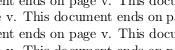A non-converging LaTeX documentBig Internet Math-Off stickers 2019

Comments in green were written by me. Comments in blue were not written by me.

Allowed HTML tags: <br> <a> <small> <b> <i> <s> <sup> <sub> <u> <spoiler> <ul> <ol> <li>
To prove you are not a spam bot, please type "emirp" backwards in the box below (case sensitive):
2018-09-13
This is a post I wrote for round 2 of The Aperiodical's Big Internet Math-Off 2018. As I went out in round 1 of the Big Math-Off, you got to read about the real projective plane instead of this.
Polynomials are very nice functions: they're easy to integrate and differentiate, it's quick to calculate their value at points, and they're generally friendly to deal with. Because of this, it can often be useful to find a polynomial that closely approximates a more complicated function.
Imagine a function defined for $$x$$ between -1 and 1. Pick $$n-1$$ points that lie on the function. There is a unique degree $$n$$ polynomial (a polynomial whose highest power of $$x$$ is $$x^n$$) that passes through these points. This polynomial is called an interpolating polynomial, and it sounds like it ought to be a pretty good approximation of the function.
So let's try taking points on a function at equally spaced values of $$x$$, and try to approximate the function:
$$f(x)=\frac1{1+25x^2}$$
Polynomial interpolations of $$\displaystyle f(x)=\frac1{1+25x^2}$$ using equally spaced points
I'm sure you'll agree that these approximations are pretty terrible, and they get worse as more points are added. The high error towards 1 and -1 is called Runge's phenomenon, and was discovered in 1901 by Carl David Tolmé Runge.
All hope of finding a good polynomial approximation is not lost, however: by choosing the points more carefully, it's possible to avoid Runge's phenomenon. Chebyshev points (named after Pafnuty Chebyshev) are defined by taking the $$x$$ co-ordinate of equally spaced points on a circle.
The following GIF shows interpolating polynomials of the same function as before using Chebyshev points.
Nice, we've found a polynomial that closely approximates the function... But I guess you're now wondering how well the Chebyshev interpolation will approximate other functions. To find out, let's try it out on the votes over time of my first round Big Internet Math-Off match.
The graphs below show the results of the match over time interpolated using 16 uniform points (left) and 16 Chebyshev points (right). You can see that the uniform interpolation is all over the place, but the Chebyshev interpolation is very close the the actual results.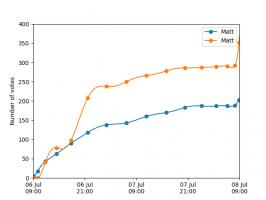Scroggs vs Parker, 6-8 July 2018, approximated using uniform points (left) and Chebyshev points (right)
But maybe you still want to see how good Chebyshev interpolation is for a function of your choice... To help you find out, I've written @RungeBot, a Twitter bot that can compare interpolations with equispaced and Chebyshev points. Just tweet it a function, and it'll show you how bad Runge's phenomenon is for that function, and how much better Chebysheb points are.
A list of constants and functions that RungeBot understands can be found here.

### Similar postsA surprising fact about quadrilateralsInteresting tautologiesBig Internet Math-Off stickers 2019Mathsteroids

Comments in green were written by me. Comments in blue were not written by me.